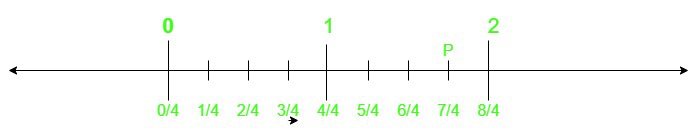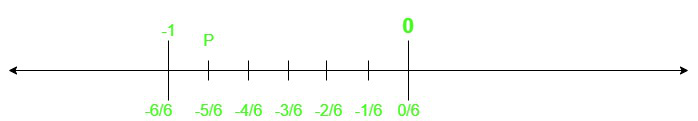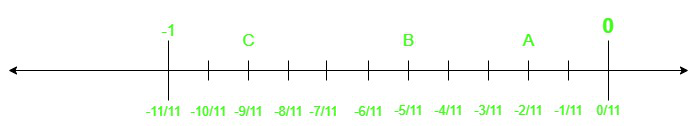Class 8 NCERT Solutions – Chapter 1 Rational Numbers – Exercise 1.2

• Last Updated : 01 Dec, 2020

Question 1. Represent these numbers on the number line?

(i) 7/4 (ii) -5/6

Solution:

(i) In number line we have to cover zero to positive integer 1 which signifies the whole no 1, after that we have to divide 1 and 2 into 4 parts and we have to cover 3 places away from 0, which denotes 3/4. And the total of seven places away from 0 represents 7/4. P represents 7/4.(ii) For representing – 5/6 we have to divide 0 to – 1 integer into 6 parts and we have to go 5 places away from 0 for – 5/6.Question 2. Represent – 2/11, -5/11, -9/11 on the number line?

Solution:

We have to divide 0 to – 1 integer into 11 parts and the distance of 2, 5, 9 from 0 towards the left of it represents – 2/11, -5/11, -9/11 marked A,  B, C,  respectively.Question 3. Write five rational numbers that are smaller than 2?

Solution:

We can write the number 2 as 6 / 3

Hence, we can write, the five rational numbers which are smaller than 2 are:

1 / 3 , 2 / 3 , 3 / 3 , 4/ 3 , 5 / 3

Question 4. Find ten rational numbers between – 2/5 and 1/2?

Solution:

For finding rational numbers between fractions we have to take L. C. M. of their denominators or its multiples. Here L. C. M. Of 5 and 2 is 10 and for finding fractions between them we have to take multiple of 10. Let us take 20 as denominator.

So,

-2 / 5 = (- 2 / 5) × (4 / 4) = -8 / 20

Also,

1 / 2 = (1 / 2)  × (10 / 10) = 10 / 20

Hence ten rational numbers between – 2 / 5 to 1 / 2 are same as rational numbers between – 8 / 20 and 10 / 20. And those are as follows

-7 / 20, -6 / 20, -5 / 20, -4 / 20, -3 / 20, -2 / 20, -1 / 20, 0, 1 / 20, 2 / 20

Question 5. Find five rational numbers between

(i) 2/3 and 4/5   (ii) – 3/2 and 5/3  (iii)1/4 and 1/2

Solution:

(i) 2 / 3 and 4 / 5

For finding rational numbers between fractions we have to take L. C. M. of their denominators or its multiples.

Here L. C. M. Of 3 and 5 is 15

And we take the denominators as multiple of 15, as 60

Hence

2 / 3 = ( 2 / 3 ) × ( 20 / 20 ) = 40 / 60

4 / 5 = ( 4 / 5 ) × ( 12 / 12 ) = 48 / 60

Five rational numbers between 2 / 3 and 4 / 5 same as five rational numbers between

40 / 60 and 48 / 60

Therefore, Five rational numbers between 40 / 60 and 48 / 60 are as follows

41 / 60,  42 / 60,  43 / 60,  44 / 60, 45 / 60

(ii) -3 / 2 and 5 / 3

Similarly,

L. C. M. of 2 and 3 is 6.

Here we take denominators same as 6.

-3 / 2 = ( -3 / 2 ) × ( 3 / 3 ) = -9 / 6

5 / 3 = ( 5 / 3 ) × ( 2 / 2 ) = 10 / 6

Hence five rational numbers between -3 / 2 and 5 / 3 are same as five rational numbers between -9 / 6 and 10 / 6 and those are  as follows

-8 / 6, -7 / 6, -1, -5 / 6, -4 / 6

(iii) 1 / 4 and 1 / 2

Here L. C. M. of 4 and 2 is 8.

Here we take denominator as multiple of 8 say 32.

Hence

1 / 4 = ( 1 / 4 ) × (8 / 8) = 8 / 32

1 / 2 = ( 1 / 2 ) × ( 16 / 16 ) = 16 / 32

Hence five rational numbers between 1 / 4 and 1 / 2 are same as five rational numbers between 8/32 and 16/32 and those are as follows

9 / 32, 10 / 32, 11 / 32, 12 / 32, 13 / 32

Question 6. Write five rational numbers greater than –2?

Solution:

We can write -2 as  -10 / 5

Hence  five rational numbers greater than -2 are as follows

-1 / 5, -2 / 5, -3 / 5, -4 / 5 ,-1

Question 7. Find ten rational numbers between 3/5 and 3/4?

Solution:

L .C. M. of 4 and 5 is 20. For finding rational number between them we should make denominator same or multiple of L .C.M.

Here we take 80.

3 / 5 = ( 3 / 5) × ( 16 / 16 ) = 48 / 80

3 / 4 = ( 3 / 4 ) × ( 20 / 20 ) = 60 / 80

Ten rational numbers between 3 / 5 and 3 / 4 are same as ten rational numbers between 48 / 80 and 60 / 80

Ten rational numbers between 48 / 80 and 60 / 80 are as follows

49 / 80, 50 / 80, 51 / 80, 52 / 80, 54 / 80, 55 / 80, 56 / 80, 57 / 80, 58 / 80, 59 / 80

My Personal Notes arrow_drop_up